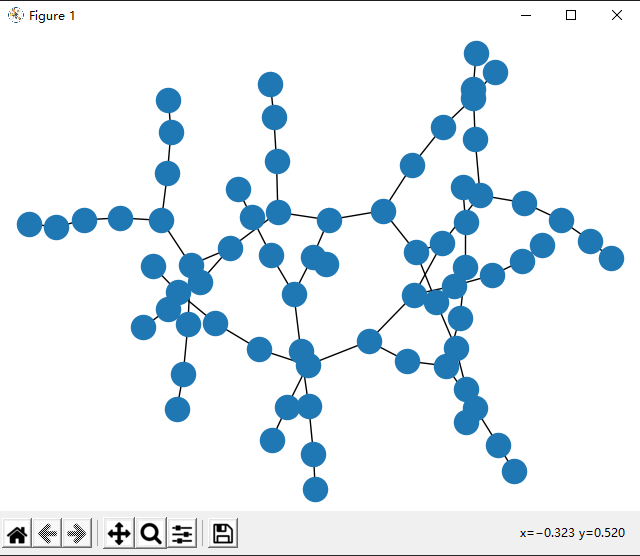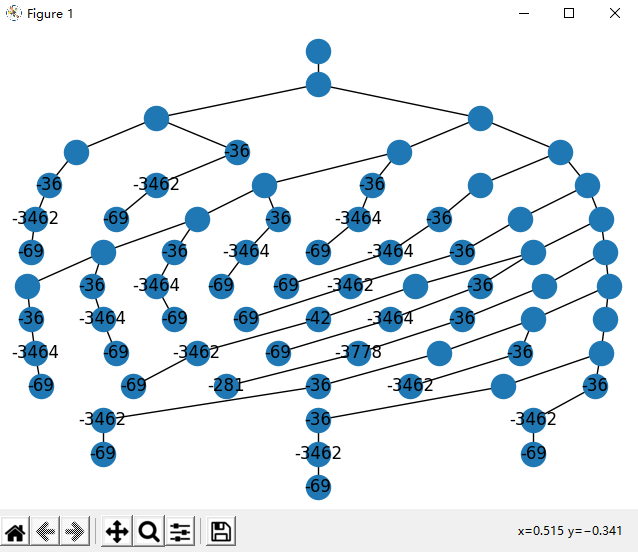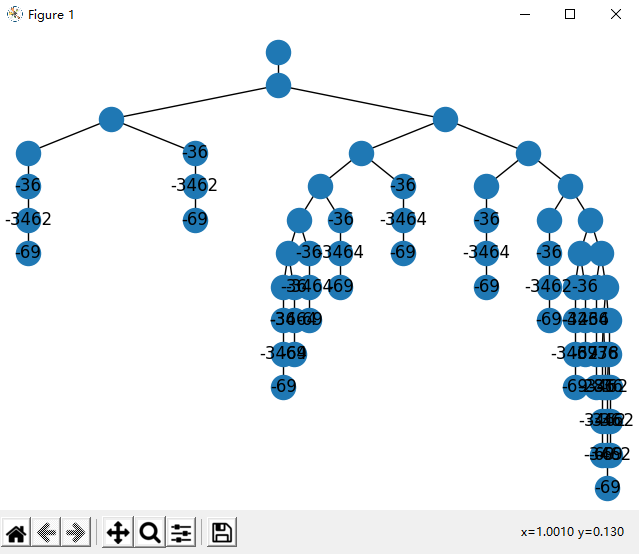# 使用networkx优雅的绘制二叉树

``````nx.draw(G)
plt.show()````````````dot_filename = str(time.time()) + '.dot'

from networkx.drawing.nx_agraph import write_dot
write_dot(G, '../dataset/pngs/' + dot_filename)

command = 'C:/"Program Files"/Graphviz/bin/dot.exe -Tpng E:/Project/dataset/pngs/' + dot_filename + \
' -o E:/WebFingerprinting/dataset/pngs/' + f.replace(".pcap", ".png")
print(command)
os.system(command)
os.remove('../dataset/pngs/' + dot_filename)````````````import networkx as nx
import random

def hierarchy_pos_ugly(G, root, levels=None, width=1., height=1.):
"""If there is a cycle that is reachable from root, then this will see infinite recursion.
G: the graph
root: the root node
levels: a dictionary
key: level number (starting from 0)
value: number of nodes in this level
width: horizontal space allocated for drawing
height: vertical space allocated for drawing"""
TOTAL = "total"
CURRENT = "current"

def make_levels(levels, node=root, currentLevel=0, parent=None):
"""Compute the number of nodes for each level
"""
if not currentLevel in levels:
levels[currentLevel] = {TOTAL: 0, CURRENT: 0}
levels[currentLevel][TOTAL] += 1
neighbors = G.neighbors(node)
for neighbor in neighbors:
if not neighbor == parent:
levels = make_levels(levels, neighbor, currentLevel + 1, node)
return levels

def make_pos(pos, node=root, currentLevel=0, parent=None, vert_loc=0):
dx = 1 / levels[currentLevel][TOTAL]
left = dx / 2
pos[node] = ((left + dx * levels[currentLevel][CURRENT]) * width, vert_loc)
levels[currentLevel][CURRENT] += 1
neighbors = G.neighbors(node)
for neighbor in neighbors:
if not neighbor == parent:
pos = make_pos(pos, neighbor, currentLevel + 1, node, vert_loc - vert_gap)
return pos

if levels is None:
levels = make_levels({})
else:
levels = {l: {TOTAL: levels[l], CURRENT: 0} for l in levels}
vert_gap = height / (max([l for l in levels]) + 1)
return make_pos({})

def hierarchy_pos_beautiful(G, root=None, width=1., vert_gap=0.2, vert_loc=0, xcenter=0.5):
'''

If the graph is a tree this will return the positions to plot this in a
hierarchical layout.

G: the graph (must be a tree)

root: the root node of current branch
- if the tree is directed and this is not given,
the root will be found and used
- if the tree is directed and this is given, then
the positions will be just for the descendants of this node.
- if the tree is undirected and not given,
then a random choice will be used.

width: horizontal space allocated for this branch - avoids overlap with other branches

vert_gap: gap between levels of hierarchy

vert_loc: vertical location of root

xcenter: horizontal location of root
'''
if not nx.is_tree(G):
raise TypeError('cannot use hierarchy_pos on a graph that is not a tree')

if root is None:
if isinstance(G, nx.DiGraph):
root = next(iter(nx.topological_sort(G)))  # allows back compatibility with nx version 1.11
else:
root = random.choice(list(G.nodes))

def _hierarchy_pos(G, root, width=1., vert_gap=0.2, vert_loc=0, xcenter=0.5, pos=None, parent=None):
'''
see hierarchy_pos docstring for most arguments

pos: a dict saying where all nodes go if they have been assigned
parent: parent of this branch. - only affects it if non-directed

'''

if pos is None:
pos = {root: (xcenter, vert_loc)}
else:
pos[root] = (xcenter, vert_loc)
children = list(G.neighbors(root))
if not isinstance(G, nx.DiGraph) and parent is not None:
children.remove(parent)
if len(children) != 0:
dx = width / len(children)
nextx = xcenter - width / 2 - dx / 2
for child in children:
nextx += dx
pos = _hierarchy_pos(G, child, width=dx, vert_gap=vert_gap,
vert_loc=vert_loc - vert_gap, xcenter=nextx,
pos=pos, parent=root)
return pos

return _hierarchy_pos(G, root, width, vert_gap, vert_loc, xcenter)``````

``````pos = networkx_tree.hierarchy_pos_beautiful(G, "Root")    # 生成树的节点位置信息，第二个参数为根节点名
node_labels = nx.get_node_attributes(G, 'attr')    # 提取树的属性标签，第二个参数为属性标签名
nx.draw(G, pos, with_labels=True, labels=node_labels)    # 绘制树
plt.show()    # 显示``````

ugly：beautiful：1. 手动狗头（

2. 楼主这布局有点丑，建议自己写个简单算法布局or使用igraph.layout的自动布局

1. @菜狗 T_T我也觉得好丑...下次再遇到二叉树可视化问题的时候我试一试
3. 你上面的搜索框用不成

1. @林阿林 是页面顶部搜索框吗？搜索不成是什么现象呢？那个是直接用原生form写的，我这边搜索功能正常
1. @kidultff 我明白了，输入文字后打回车可以搜索，但点击那个搜索图标，没反应
1. @林阿林 看了一下，设计确实反人类，目前已修复~